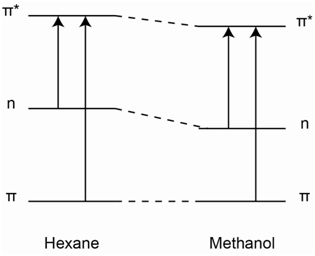# 2.4: Effect of Solvent

•• Thomas Wenzel
• Bates College
$$\newcommand{\vecs}{\overset { \rightharpoonup} {\mathbf{#1}} }$$ $$\newcommand{\vecd}{\overset{-\!-\!\rightharpoonup}{\vphantom{a}\smash {#1}}}$$$$\newcommand{\id}{\mathrm{id}}$$ $$\newcommand{\Span}{\mathrm{span}}$$ $$\newcommand{\kernel}{\mathrm{null}\,}$$ $$\newcommand{\range}{\mathrm{range}\,}$$ $$\newcommand{\RealPart}{\mathrm{Re}}$$ $$\newcommand{\ImaginaryPart}{\mathrm{Im}}$$ $$\newcommand{\Argument}{\mathrm{Arg}}$$ $$\newcommand{\norm}{\| #1 \|}$$ $$\newcommand{\inner}{\langle #1, #2 \rangle}$$ $$\newcommand{\Span}{\mathrm{span}}$$ $$\newcommand{\id}{\mathrm{id}}$$ $$\newcommand{\Span}{\mathrm{span}}$$ $$\newcommand{\kernel}{\mathrm{null}\,}$$ $$\newcommand{\range}{\mathrm{range}\,}$$ $$\newcommand{\RealPart}{\mathrm{Re}}$$ $$\newcommand{\ImaginaryPart}{\mathrm{Im}}$$ $$\newcommand{\Argument}{\mathrm{Arg}}$$ $$\newcommand{\norm}{\| #1 \|}$$ $$\newcommand{\inner}{\langle #1, #2 \rangle}$$ $$\newcommand{\Span}{\mathrm{span}}$$$$\newcommand{\AA}{\unicode[.8,0]{x212B}}$$

## The peaks in the 320-380 nm portion of the UV absorption spectrum of pyridine shift noticeably toward the blue (high energy) portion of the spectrum on changing the solvent from hexane to methanol. Account for this change.

These are the lowest energy peaks in the spectrum and correspond to the n-$$\pi$$* transition in pyridine. Hexane (C6H14) is a non-polar hydrocarbon. Methanol (CH3OH) is a polar solvent with the ability to form hydrogen bonds. For pyridine, the hydrogen atom of the hydroxyl group of methanol will form hydrogen bonds with the lone pair on the nitrogen atoms, as shown in Figure $$\PageIndex{22}$$. Hexane cannot form such hydrogen bonds.Figure $$\PageIndex{22}$$. Hydrogen bond between methanol and pyridine.

In order to account for the blue-shift in the spectrum, we need to consider what, if anything, will happen to the energies of the n, $$\pi$$, and $$\pi$$* orbitals. Bonding between two atomic orbitals leads to the formation of a bonding and anti-bonding molecular orbital, one of which drops in energy and the other of which rises in energy. The electrostatic attraction between a positively charged hydrogen atom and negatively charged lone pair of electrons in a hydrogen-bond (as illustrated in Figure $$\PageIndex{22}$$ for methanol and pyridine) is a stabilizing interaction. Therefore, the energy of the non-bonding electrons will be lowered.

The picture in Figure $$\PageIndex{23}$$ shows representations of a $$\pi$$- and $$\pi$$*-orbital.Figure $$\PageIndex{23}$$. Representations of the $$\pi$$- and $$\pi$$*-molecular orbitals.

Electrons in a ­$$\pi$$-orbital may be able to form a weak dipole-dipole interaction with the hydroxyl hydrogen atom of methanol. This weak interaction may cause a very slight drop in energy of the $$\pi$$-orbital, but it will not be nearly as pronounced as that of the non-bonding electrons. Similarly, if an electron has been excited to the $$\pi$$*-orbital, it has the ability to form a weak dipole-dipole interaction with the hydroxyl hydrogen atom of methanol. This weak interaction will cause a drop in energy of the $$\pi$$*-orbital, but it will not be nearly as pronounced as that of the non-bonding electrons. However, the drop in energy of the $$\pi$$*-orbital will be larger than that of the $$\pi$$-orbital because the $$\pi$$*-orbital points out from the C=C bond and is more accessible to interact with the hydroxyl hydrogen atom of methanol than the $$\pi$$-orbital. The diagram in Figure $$\PageIndex{24}$$ shows the relative changes in the energies of the n, $$\pi$$, and $$\pi$$* orbitals that would occur on changing the solvent from hexane to methanol with stabilization occurring in the order n > $$\pi$$* > $$\pi$$.Figure $$\PageIndex{24}$$. Relative changes in energies of the n, $$\pi$$, and $$\pi$$* orbitals that occurs on changing the solvent from hexane to methanol.

An examination of the relative energies between hexane and methanol shows that both the n and $$\pi$$* levels drop in energy, but the drop of the n level is greater than the drop of the $$\pi$$* level. Therefore, the n-$$\pi$$* transition moves to higher energy, hence a blue-shift is observed in the peaks in the spectrum in the 320-380 nm range of pyridine. The blue-shift that is observed is referred to as a hypsochromic shift.

## The peaks in the UV spectrum of benzene shift slightly toward the red (low energy) portion of the spectrum on changing the solvent from hexane to methanol. Account for this change.

The absorption in benzene corresponds to the $$\pi$$-$$\pi$$* transition. Using the diagram in Figure $$\PageIndex{24}$$, the drop in energy of the $$\pi$$*-orbital is more than that of the $$\pi$$-orbital. Therefore, the $$\pi$$-$$\pi$$* transition is slightly lower in energy and the peaks shift toward the red. The red-shift is referred to as a bathochromic shift.

Note as well that the change in the position of the peak for the $$\pi$$-$$\pi$$* transition of benzene would be less than that for the n-$$\pi$$* transition of pyridine because the stabilization of the non-bonding electrons is greater than the stabilization of the electrons in the $$\pi$$*-orbital.

This page titled 2.4: Effect of Solvent is shared under a CC BY-NC 4.0 license and was authored, remixed, and/or curated by Thomas Wenzel via source content that was edited to the style and standards of the LibreTexts platform; a detailed edit history is available upon request.